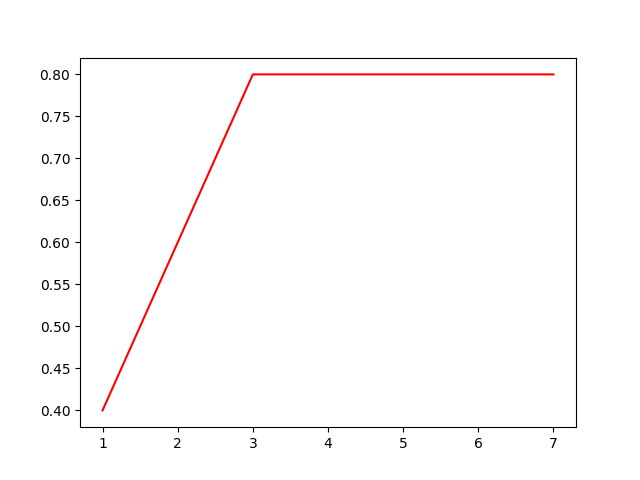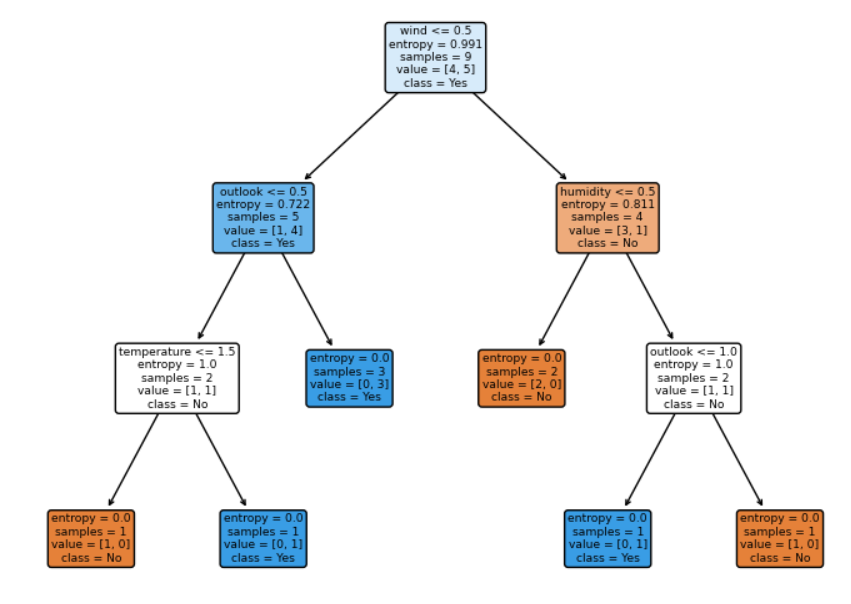# Decision Tree by Python

wuchangjian2021-11-05 14:43:14编程学习

Part1.Introduce

This article records the process of building a decision tree(ID3) which is my first curriculum design.

The work I need to do is that load and process the data first, then use the train data to build the decision tree and finally use the test data to predict if people can go out to play.

The data set:

 id outlook temperature humidity wind play 1 sunny hot high weak no 2 sunny hot high strong no 3 overcast hot high weak yes 4 rainy mild high weak yes 5 rainy cool normal weak yes 6 rainy cool normal strong no 7 overcast cool normal strong yes 8 sunny mild high weak no 9 sunny cool normal weak yes 10 rainy mild normal weak yes 11 sunny mild normal strong yes 12 overcast mild high strong yes 13 overcast hot normal weak yes 14 rainy mild high strong no

In this data set, there are 4 kinds of features where every one contains element that varying quantities. I will use entropy and gini to build the tree.

Part2.Implementation

I use the txt type to save file so that the first step is to load data into narray type and reshape the data.

``````'''
function： load txt file
input：the txt file path
output：the data(narray) in txt file
'''
data=[]
feature=[]
filepath = path
f = open(filepath,'r')  # f -> string
f_data = f.readlines()  # f_data -> list
for row in f_data:
row = row.strip('\n')
data.append(row.split(' '))
for i in range(len(data)):
for j in data[i]:
feature.append(j)
array = np.array(feature)
array = array.reshape(len(f_data),int(array.size/len(f_data)))
return array

# # read and process data
file_path = 'play.txt'
words = Myread_data(file_path)  # get data``````

2. precess data:

Because the data name are string type, now I use a function that I write to turn the feature name into number type.

``````'''
function: 1.turn features(words) into numbers
2.get off the feature labels
input: data(words)
output: features(numbers)
'''
def Myword2num(word):
dic = {}
data = word
data = data[1:,1:]
for j in range(data.shape):
count = 0
for i in range(data.shape):
if data[i,j] not in dic:
dic[data[i,j]] = count
count += 1
for j in range(data.shape):
for i in range(data.shape):
if data[i,j] in dic:
data[i,j] = dic[data[i,j]]
return data

features = Myword2num(words)[:,:-1] # divide the data into features and labels
labels = Myword2num(words)[:,-1]``````

By the way, I add the features name and labels name and  devide the data into train data and test data(both contain features and labels).

``````feature_names = words[0,1:-1]  # ['outlook' 'temperature' 'humidity' 'wind']
label_names = ['No', 'Yes']
X_train,X_test,Y_train,Y_test=train_test_split(features,labels,test_size=0.3)  # divide the data into train and test set``````

3. build decision tree

the sklearn library provide some functions to help user build tree:

1)DecisionTreeClassifier():

criterion: chose method entrop or gini to make decisions about how to divide data.

random_state: something like  randomseed which is used to keep model stable.

splitter: 'best' means the tree branch in favor of more important features when                                 making decisions, while 'random' means more random when making                                 decisions.

max_depth: the train depth in every train loop.

2)fit(): fitting the features and labels

3)score():use the test data to grade the model, the score closer to 1 the better the                         model is.

In this train, I use a 7 times loop, that the max_depth +1 in every new loop, I think it could help make the model more fitting.

``````# # build the decision tree
test_score = []  # save the score for every train
# train 7 times
# the depth(from 1) +1 every time
for i in range(7):
clf=tree.DecisionTreeClassifier(criterion="entropy"
,random_state=30
,splitter='best'
,max_depth=i+1
)
clf=clf.fit(X_train,Y_train) # fit features and labels
score=clf.score(X_test,Y_test)  # get the test score which is better when close to 1
test_score.append(score)``````

4. show the tree and scores

Learned from the last section, there are 7 loop which means there are 7 scores. I keep the scores and use the plt function to draw them. Meanwhile I use the plot_tree function to draw the decision tree in the final train.

``````# # show the train scores
plt.plot(range(1,8),test_score,color="red",label="max_depth")  # show the scores with every train
plt.show()

# # show the decision tree
tree.plot_tree(clf,
feature_names=feature_names,
class_names = label_names,
filled=True,
rounded=True)
plt.show()``````

5. results analysis

The scores:As we can see, in the 7 loops, the score in loop 1 and 2 are smaller, which means they have a poor effect. Meanwhile the other scores are all 0.8. Now let's see the final tree.Obviousily, the features wind contain more specific gravity, at the second layer, the entropy of outlook and humidity are same, and it's strange that the third lay contains another outlook, because the element in it has different entropy.

### 被告人张宁宁等人开设赌场、非法经营案一审开庭

2022-08-12 19:37:47 2022年8月10日至12日，...

### 中央气象台15日6时继续发布高温红色预警

2022-08-15 09:42:48 据中央气象台网站消息，中央气象...

### “全网专钓小鲜女”惹争议，君乐宝悦鲜活致歉

2022-08-26 21:30:10 近日，君乐宝悦鲜活牛奶的一则广...

### 简单了解------网络搭建

#### 发表评论◎欢迎参与讨论，请在这里发表您的看法、交流您的观点。